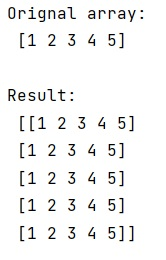# Concatenate (or clone) a NumPy array N times

Learn, how to Concatenate (or clone) a NumPy array N times?
Submitted by Pranit Sharma, on January 13, 2023

NumPy is an abbreviated form of Numerical Python. It is used for different types of scientific operations in python. Numpy is a vast library in python which is used for almost every kind of scientific or mathematical operation. It is itself an array which is a collection of various methods and functions for processing the arrays.

## NumPy - Concatenate (or clone) an array N times

Suppose we need to work on a MxN NumPy matrix by cloning an Mx1 ndarray N times, we need to find an appropriate approach for this.

For this purpose, we first need to define a value of N, and then we will create a NumPy array. Now we will use numpy.vstack() method. This method stacks arrays in sequence vertically (row wise).

This is equivalent to concatenation along the first axis after 1-D arrays of shape (N,) have been reshaped to (1, N).
This function makes the most sense for arrays with up to 3 dimensions. For instance, for pixel-data with a height (first axis), width (second axis), and r/g/b channels (third axis). The functions concatenate, stack and block provide more general stacking and concatenation operations.

Let us understand with the help of an example,

## Python code to concatenate (or clone) a NumPy array N times

```# Import numpy
import numpy as np

# Creating a numpy array
arr = np.array([1,2,3,4,5])

# Display original array
print("Orignal array:\n",arr,"\n")

# Defining a value of N
N = 5

# Using vstack method
res = np.vstack([arr]*N)

# Display result
print("Result:\n",res,"\n")
```

Output: